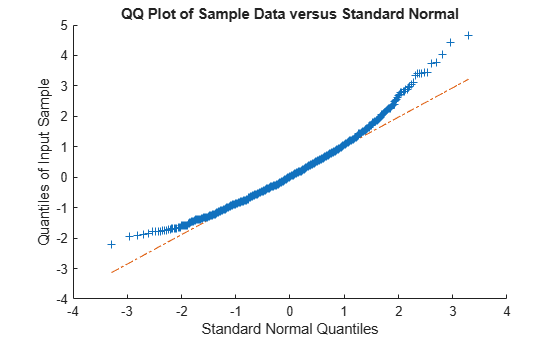# johnsrnd

Johnson system random numbers

## Syntax

```r = johnsrnd(quantiles,m,n) r = johnsrnd(quantiles) [r,type] = johnsrnd(...) [r,type,coefs] = johnsrnd(...) ```

## Description

`r = johnsrnd(quantiles,m,n)` returns an `m`-by-`n` matrix of random numbers drawn from the distribution in the Johnson system that satisfies the quantile specification given by `quantiles`. `quantiles` is a four-element vector of quantiles for the desired distribution that correspond to the standard normal quantiles [–1.5 –0.5 0.5 1.5]. In other words, you specify a distribution from which to draw random values by designating quantiles that correspond to the cumulative probabilities [0.067 0.309 0.691 0.933]. `quantiles` may also be a `2`-by-`4` matrix whose first row contains four standard normal quantiles, and whose second row contains the corresponding quantiles of the desired distribution. The standard normal quantiles must be spaced evenly.

Note

Because `r` is a random sample, its sample quantiles typically differ somewhat from the specified distribution quantiles.

`r = johnsrnd(quantiles)` returns a scalar value.

`r = johnsrnd(quantiles,m,n,...)` or ```r = johnsrnd(quantiles,[m,n,...])``` returns an `m`-by-`n`-by-... array.

`[r,type] = johnsrnd(...)` returns the type of the specified distribution within the Johnson system. `type` is `'SN'`, `'SL'`, `'SB'`, or `'SU'`. Set `m` and `n` to zero to identify the distribution type without generating any random values.

The four distribution types in the Johnson system correspond to the following transformations of a normal random variate:

• `'SN'` — Identity transformation (normal distribution)

• `'SL'` — Exponential transformation (lognormal distribution)

• `'SB'` — Logistic transformation (bounded)

• `'SU'` — Hyperbolic sine transformation (unbounded)

`[r,type,coefs] = johnsrnd(...)` returns coefficients `coefs` of the transformation that defines the distribution. `coefs` is ```[gamma, eta, epsilon, lambda]```. If `z` is a standard normal random variable and `h` is one of the transformations defined above, `r = lambda*h((z-gamma)/eta)+epsilon` is a random variate from the distribution type corresponding to `h`.

## Examples

collapse all

This example shows several different approaches to using the Johnson system of flexible distribution families to generate random numbers and fit a distribution to sample data.

Generate random values with longer tails than a standard normal.

```rng default; % For reproducibility r = johnsrnd([-1.7 -.5 .5 1.7],1000,1); figure; qqplot(r);```Generate random values skewed to the right.

```r = johnsrnd([-1.3 -.5 .5 1.7],1000,1); figure; qqplot(r);```Generate random values that match some sample data well in the right-hand tail.

```load carbig; qnorm = [.5 1 1.5 2]; q = quantile(Acceleration, normcdf(qnorm)); r = johnsrnd([qnorm;q],1000,1); [q;quantile(r,normcdf(qnorm))]```
```ans = 2×4 16.7000 18.2086 19.5376 21.7263 16.6986 18.2220 19.9078 22.0918 ```

Determine the distribution type and the coefficients.

`[r,type,coefs] = johnsrnd([qnorm;q],0)`
```r = [] ```
```type = 'SU' ```
```coefs = 1×4 1.0920 0.5829 18.4382 1.4494 ```